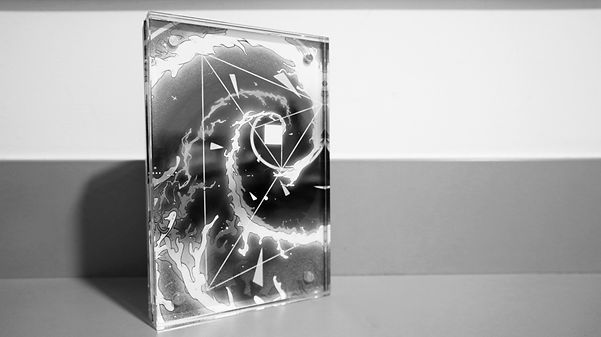top of page# 42

"The most incomprehensible thing about the world is that it is comprehensible." ——Albert Einstein

Every picture in the illustration part is a question. Including mathematics, physics, chemistry, astronomy, etc., the art works ask: "why our world can be calculated". For example, why the Pythagorean theorem is a2+b2=c2, why is the golden ratio 0.618, why π is 3.14, why Euler's formula can be associated with several constants, why Maxwell's equations can unify Light, electricity, magnetism, why Mendeleev's periodic table inspiration come from dreams and so on.

2020.10.bottom of page